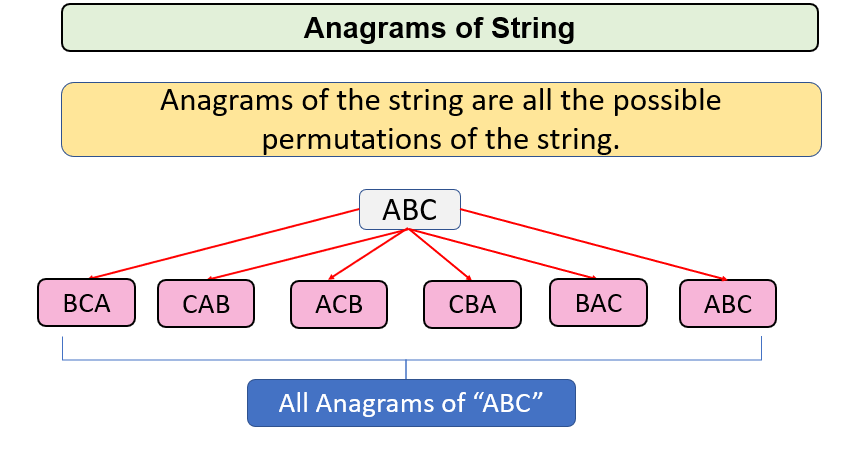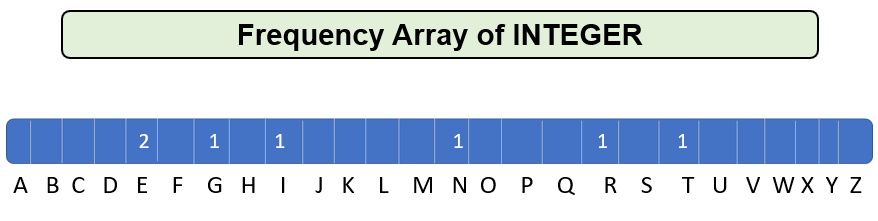# Check if two Strings are anagrams of each other

Problem Statement: Given two strings, check if two strings are anagrams of each other or not.

Examples:

```Example 1:
Input: CAT, ACT
Output: true
Explanation: Since the count of every letter of both strings are equal.

Example 2:
Input: RULES, LESRT
Output: false
Explanation: Since the count of U and T  is not equal in both strings.
```

## Solution

Disclaimer: Don’t jump directly to the solution, try it out yourself first.Solution 1:

Approach: Sort both the string and compare each and every letter of both strings. If all letters matched then, print true. Otherwise, print false.

For Eg.

We have

Str1 = “INTEGER”

Str2=”TEGERNI”

After sorting Str1 and Str2, we find that both of the strings are

Str1 =” EEGINRT”

Str2=” EEGINRT”

Since both of the strings are the same, this means both Str1 and Str2 are anagrams of each other.

Code:

## C++ Code

``````#include <iostream>
#include <algorithm>
using namespace std;
bool CheckAnagrams(string str1, string str2)
{
// Case 1: when both of the strings have different lengths
if (str1.length() != str2.length())
return false;

sort(str1.begin(), str1.end());
sort(str2.begin(), str2.end());

// Case 2: check if every character of str1 and str2 matches with each other
for (int i = 0; i < str1.length(); i++)
{
if (str1[i] != str2[i])
return false;
}
return true;
}
int main()
{
string Str1 = "INTEGER";
string Str2 = "TEGERNI";
if(CheckAnagrams(Str1, Str2))
cout << "True" << endl;
else
cout<<"False"<<endl;
return 0;
}
``````

Output: True

Time Complexity: O(nlogn) since sorting function requires nlogn iterations.

Space Complexity: O(1)

## Java Code

``````import java.util.*;
public class Main
{
public static String SortString(String str)
{
char c[] = str.toCharArray();
Arrays.sort(c);
return new String(c);
}
public static boolean checkAnagrams(String str1, String str2)
{
// Case 1: when both of the strings have different lengths
if (str1.length() != str2.length())
return false;

str1 = SortString(str1);
str2 = SortString(str2);

// Case 2: check if every character of str1 and str2 matches with each other
for (int i = 0; i < str1.length(); i++)
{
if (str1.charAt(i) != str2.charAt(i))
return false;
}
return true;
}
public static void main(String args[])
{
String Str1 = "INTEGER";
String Str2 = "TEGERNI";
System.out.println(checkAnagrams(Str1, Str2));
}
}
``````

Output: True

Time Complexity: O(nlogn) since sorting function requires nlogn iterations.

Space Complexity: O(1)

Solution 2:

Approach: Just count the frequency of every element in Str1 and iterate through Str2 and decrease the count of every element in the frequency array. Now iterate again, if the frequency at any point is not 0 this means, strings are not anagrams of each other.

For Eg,

Str1 = ”INTEGER”

Str2 = ”NTEGERI”

Frequency array of every element :We check for every element of Str2 and find that all elements are found, so return true.

Code:

## C++ Code

``````#include <iostream>
#include <algorithm>
using namespace std;
bool CheckAnagrams(string str1, string str2)
{
// when both of the strings have different lengths
if (str1.length() != str2.length())
return false;

int freq = {0};
for (int i = 0; i < str1.length(); i++)
{
freq[str1[i] - 'A']++;
}
for (int i = 0; i < str2.length(); i++)
{
freq[str2[i] - 'A']--;
}
for (int i = 0; i < 26; i++)
{
if (freq[i] != 0)
return false;
}
return true;
}
int main()
{
string Str1 = "INTEGER";
string Str2 = "TEGERNI";
if(CheckAnagrams(Str1, Str2))
cout << "True" << endl;
else
cout<<"False"<<endl;
return 0;
}
``````

Output: True

Time Complexity: O(n) where n is the length of string

Space Complexity: O(1)

## Java Code

``````import java.util.*;
public class Main {
public static boolean checkAnagrams(String str1, String str2) {
// when both of the strings have different lengths
if (str1.length() != str2.length())
return false;

int[] freq = new int;
for (int i = 0; i < str1.length(); i++) {
freq[str1.charAt(i) - 'A']++;
}
for (int i = 0; i < str2.length(); i++) {
freq[str2.charAt(i) - 'A']--;
}
for (int i = 0; i < 26; i++) {
if (freq[i] != 0)
return false;
}
return true;
}
public static void main(String args[]) {
String Str1 = "INTEGER";
String Str2 = "TEGERNI";
System.out.println(checkAnagrams(Str1, Str2));
}
}
``````

Output: true

Time Complexity: O(n) where n is the length of string

Space Complexity: O(1)

Special thanks to Gurmeet Singh for contributing to this article on takeUforward. If you also wish to share your knowledge with the takeUforward fam, please check out this article